Test: Asymptotic Worst Case Time & Space Complexity- 2

# Test: Asymptotic Worst Case Time & Space Complexity- 2 - Computer Science Engineering (CSE)

Test Description

## 20 Questions MCQ Test GATE Computer Science Engineering(CSE) 2023 Mock Test Series - Test: Asymptotic Worst Case Time & Space Complexity- 2

Test: Asymptotic Worst Case Time & Space Complexity- 2 for Computer Science Engineering (CSE) 2023 is part of GATE Computer Science Engineering(CSE) 2023 Mock Test Series preparation. The Test: Asymptotic Worst Case Time & Space Complexity- 2 questions and answers have been prepared according to the Computer Science Engineering (CSE) exam syllabus.The Test: Asymptotic Worst Case Time & Space Complexity- 2 MCQs are made for Computer Science Engineering (CSE) 2023 Exam. Find important definitions, questions, notes, meanings, examples, exercises, MCQs and online tests for Test: Asymptotic Worst Case Time & Space Complexity- 2 below.
Solutions of Test: Asymptotic Worst Case Time & Space Complexity- 2 questions in English are available as part of our GATE Computer Science Engineering(CSE) 2023 Mock Test Series for Computer Science Engineering (CSE) & Test: Asymptotic Worst Case Time & Space Complexity- 2 solutions in Hindi for GATE Computer Science Engineering(CSE) 2023 Mock Test Series course. Download more important topics, notes, lectures and mock test series for Computer Science Engineering (CSE) Exam by signing up for free. Attempt Test: Asymptotic Worst Case Time & Space Complexity- 2 | 20 questions in 60 minutes | Mock test for Computer Science Engineering (CSE) preparation | Free important questions MCQ to study GATE Computer Science Engineering(CSE) 2023 Mock Test Series for Computer Science Engineering (CSE) Exam | Download free PDF with solutions
 1 Crore+ students have signed up on EduRev. Have you?
Test: Asymptotic Worst Case Time & Space Complexity- 2 - Question 1

### A list of n string, each of length n, is sorted into lexicographic order using the merge-sort algorithm. The worst case running time of this computation is

Detailed Solution for Test: Asymptotic Worst Case Time & Space Complexity- 2 - Question 1

The recurrence tree for merge sort will have height Log(n). And O(n^2) work will be done at each level of the recurrence tree (Each level involves n comparisons and a comparison takes O(n) time in worst case). So time complexity of this Merge Sort will be [Tex]O (n^2 log n) [/Tex].

Test: Asymptotic Worst Case Time & Space Complexity- 2 - Question 2

### In quick sort, for sorting n elements, the (n/4)th smallest element is selected as pivot using an O(n) time algorithm. Q. What is the worst case time complexity of the quick sort?

Detailed Solution for Test: Asymptotic Worst Case Time & Space Complexity- 2 - Question 2

The recursion expression becomes: T(n) = T(n/4) + T(3n/4) + cn After solving the above recursion, we get  θ(nLogn).

Test: Asymptotic Worst Case Time & Space Complexity- 2 - Question 3

### Consider the Quicksort algorithm. Suppose there is a procedure for finding a pivot element which splits the list into two sub-lists each of which contains at least one-fifth of the elements. Let T(n) be the number of comparisons required to sort n elements. Then

Detailed Solution for Test: Asymptotic Worst Case Time & Space Complexity- 2 - Question 3

For the case where n/5 elements are in one subset, T(n/5) comparisons are needed for the first subset with n/5 elements, T(4n/5) is for the rest 4n/5 elements, and n is for finding the pivot. If there are more than n/5 elements in one set then other set will have less than 4n/5 elements and time complexity will be less than T(n/5) + T(4n/5) + n because recursion tree will be more balanced.

Test: Asymptotic Worst Case Time & Space Complexity- 2 - Question 4

Consider the following functions:

f(n) = 2^n
g(n) = n!
h(n) = n^logn

Q. Which of the following statements about the asymptotic behavior of f(n), g(n), and h(n) is true?

Detailed Solution for Test: Asymptotic Worst Case Time & Space Complexity- 2 - Question 4

According to order of growth: h(n) < f(n) < g(n) (g(n) is asymptotically greater than f(n) and f(n) is asymptotically greater than h(n) ) We can easily see above order by taking logs of the given 3 functions

lognlogn < n < log(n!) (logs of the given f(n), g(n) and h(n)).

Note that log(n!) =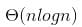Test: Asymptotic Worst Case Time & Space Complexity- 2 - Question 5

In the following C function, let n >= m.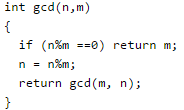Q. How many recursive calls are made by this function?

Detailed Solution for Test: Asymptotic Worst Case Time & Space Complexity- 2 - Question 5

Above code is implementation of the Euclidean algorithm for finding Greatest Common Divisor (GCD).

Test: Asymptotic Worst Case Time & Space Complexity- 2 - Question 6

Which of the following sorting algorithms has the lowest worst-case complexity?

Detailed Solution for Test: Asymptotic Worst Case Time & Space Complexity- 2 - Question 6

Worst case complexities for the above sorting algorithms are as follows: Merge Sort — nLogn Bubble Sort — n^2 Quick Sort — n^2 Selection Sort — n^2

Test: Asymptotic Worst Case Time & Space Complexity- 2 - Question 7

Consider the following functions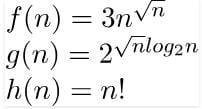Detailed Solution for Test: Asymptotic Worst Case Time & Space Complexity- 2 - Question 7

g(n) =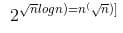f(n) and g(n) are of same asymptotic order and following statements are true. f(n) = O(g(n)) g(n) = O(f(n)). (a) and (b) are false because n! is of asymptotically higher order than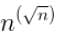.

Test: Asymptotic Worst Case Time & Space Complexity- 2 - Question 8

Consider the following three claims I (n + k)^m = θ(n^m), where k and m are constants II 2^(n + 1) = 0(2^n) III 2^(2n + 1) = 0(2^n) Which of these claims are correct?

Detailed Solution for Test: Asymptotic Worst Case Time & Space Complexity- 2 - Question 8

(I) (n+m)^k = n^k + c1*n^(k-1) + ... k^m = θ(n^k)
(II) 2^(n+1) = 2*2^n = θ(2^n)

Test: Asymptotic Worst Case Time & Space Complexity- 2 - Question 9

Let s be a sorted array of n integers. Let t(n) denote the time taken for the most efficient algorithm to determined if there are two elements with sum less than 1000 in s. which of the following statements is true?

Detailed Solution for Test: Asymptotic Worst Case Time & Space Complexity- 2 - Question 9

Let array be sorted in ascending order, if sum of first two elements is less than 1000 then there are  two elements with sum less than 1000 otherwise not. For array sorted in descending order we need to check last two elements. For an array data structure, number of operations are fixed in both the cases and not dependent on n, complexity is O(1)

Test: Asymptotic Worst Case Time & Space Complexity- 2 - Question 10

Consider the following function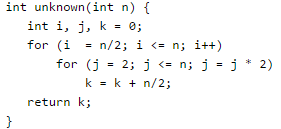Q. What is the returned value of the above function?

Detailed Solution for Test: Asymptotic Worst Case Time & Space Complexity- 2 - Question 10

The outer loop runs n/2 or θ times. The inner loop runs θ(Logn) times (Note that j is multiplied by 2 in every iteration). So the statement "k = k + n/2;" runs θ(nLogn) times. The statement increases value of k by n/2. So the value of k becomes n/2* θ(nLogn) which is θ(n^2Logn)

Test: Asymptotic Worst Case Time & Space Complexity- 2 - Question 11

The number of elements that can be sorted in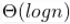time using heap sort is

Detailed Solution for Test: Asymptotic Worst Case Time & Space Complexity- 2 - Question 11

Time complexity of Heap Sort is θ(mLogm) for m input elements. For m =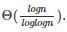, the value of θ(m * Logm) will be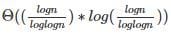which will be [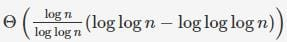which is θ(Log n)

Test: Asymptotic Worst Case Time & Space Complexity- 2 - Question 12

Consider the following two functions. What are time complexities of the functions?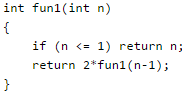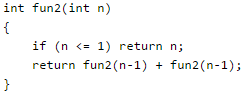Detailed Solution for Test: Asymptotic Worst Case Time & Space Complexity- 2 - Question 12

Time complexity of fun1() can be written as T(n) = T(n-1) + C which is O(n) Time complexity of fun2() can be written as T(n) = 2T(n-1) + C which is O(2^n)

Test: Asymptotic Worst Case Time & Space Complexity- 2 - Question 13

In quick sort, for sorting n elements, the (n/4)th smallest element is selected as pivot using an O(n) time algorithm. What is the worst case time complexity of the quick sort?

Detailed Solution for Test: Asymptotic Worst Case Time & Space Complexity- 2 - Question 13

Answer(B) The recursion expression becomes: T(n) = T(n/4) + T(3n/4) + cn After solving the above recursion, we get heta(nLogn).

Test: Asymptotic Worst Case Time & Space Complexity- 2 - Question 14

Consider the Quicksort algorithm. Suppose there is a procedure for finding a pivot element which splits the list into two sub-lists each of which contains at least one-fifth of the elements. Let T(n) be the number of comparisons required to sort n elements. Then

Detailed Solution for Test: Asymptotic Worst Case Time & Space Complexity- 2 - Question 14

For the case where n/5 elements are in one subset, T(n/5) comparisons are needed for the first subset with n/5 elements, T(4n/5) is for the rest 4n/5 elements, and n is for finding the pivot. If there are more than n/5 elements in one set then other set will have less than 4n/5 elements and time complexity will be less than T(n/5) + T(4n/5) + n because recursion tree will be more balanced.

Test: Asymptotic Worst Case Time & Space Complexity- 2 - Question 15

Consider the following segment of C-code: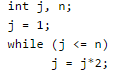Q. The number of comparisons made in the execution of the loop for any n > 0 is: Base of Log is 2 in all options.

Detailed Solution for Test: Asymptotic Worst Case Time & Space Complexity- 2 - Question 15

We can see it by taking few examples like n = 1, n = 3, etc.

For example, for n=5 we have the following (4) comparisons: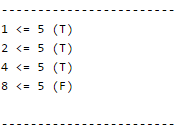CEIL(log_2 n)+2 = CEIL(log_2 5) + 2 = CEIL(2.3) + 2 = 3 + 2 = 5

Test: Asymptotic Worst Case Time & Space Complexity- 2 - Question 16

Consider the following C-program fragment in which i, j and n are integer variables.

for (i = n, j = 0; i >0; i /= 2, j += i);

Q. Let val(j) denote the value stored in the variable j after termination of the for loop. Which one of the following is true?

Detailed Solution for Test: Asymptotic Worst Case Time & Space Complexity- 2 - Question 16

The variable j is initially 0 and value of j is sum of values of i. i is initialized as n and is reduced to half in each iteration. j = n/2 + n/4 + n/8 + .. + 1 = Θ(n) Note the semicolon after the for loop, so there is nothing in the body.

Test: Asymptotic Worst Case Time & Space Complexity- 2 - Question 17

The minimum number of comparisons required to find the minimum and the maximum of 100 numbers is _________________.

Detailed Solution for Test: Asymptotic Worst Case Time & Space Complexity- 2 - Question 17

To find minimum and maximum element out of n numbers, we need to have at least (3n/2-2) comparisons.

Test: Asymptotic Worst Case Time & Space Complexity- 2 - Question 18

Consider the following pseudo code. What is the total number of multiplications to be performed?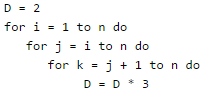Test: Asymptotic Worst Case Time & Space Complexity- 2 - Question 19

You have an array of n elements. Suppose you implement quicksort by always choosing the central element of the array as the pivot. Then the tightest upper bound for the worst case performance is

Detailed Solution for Test: Asymptotic Worst Case Time & Space Complexity- 2 - Question 19

The central element may always be an extreme element, therefore time complexity in worst case becomes O(n2)

Test: Asymptotic Worst Case Time & Space Complexity- 2 - Question 20

Consider the following C-function: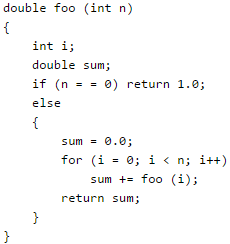Q. The space complexity of the above function is:

Detailed Solution for Test: Asymptotic Worst Case Time & Space Complexity- 2 - Question 20

Note that the function foo() is recursive. Space complexity is O(n) as there can be at most O(n) active functions (function call frames) at a time.

## GATE Computer Science Engineering(CSE) 2023 Mock Test Series

151 docs|215 tests
Information about Test: Asymptotic Worst Case Time & Space Complexity- 2 Page
In this test you can find the Exam questions for Test: Asymptotic Worst Case Time & Space Complexity- 2 solved & explained in the simplest way possible. Besides giving Questions and answers for Test: Asymptotic Worst Case Time & Space Complexity- 2, EduRev gives you an ample number of Online tests for practice

## GATE Computer Science Engineering(CSE) 2023 Mock Test Series

151 docs|215 tests(Scan QR code)